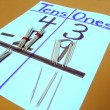### Blog Archives

•### Math - Popsicle Stick Regrouping Fun

Objectives: Learning and practising regrouping Print examples of regrouping in student math journals Learn term names:  minuend take away subtrahend equals difference/answer (for subtraction) and addend plus addend equals sum/answer (for addition) Materials for each group … View Full Lesson →

• ### Math - Toothpick Art/Math Shapes

Objectives: review primary colors practise using ruler to make straight lines make simple shapes, at least as large as a toothpick:  triangles, squares, rectangles review/use math words: parallel, perpendicular, horizontal, vertical, diagonal reinforcement of math shape … View Full Lesson →